## How to count unique values based on multiple criteria in Excel?

This article, I will take some examples for you to count unique values based on one or more criteria in a worksheet. The following detailed steps may help you.

Count unique values based on one criteria

Count unique values based on two given dates

Count unique values based on two criteria

Count unique values based on three criteria

####Count unique values based on one criteria

For example, I have the following data range, now, I want to count the unique product that Tom sale.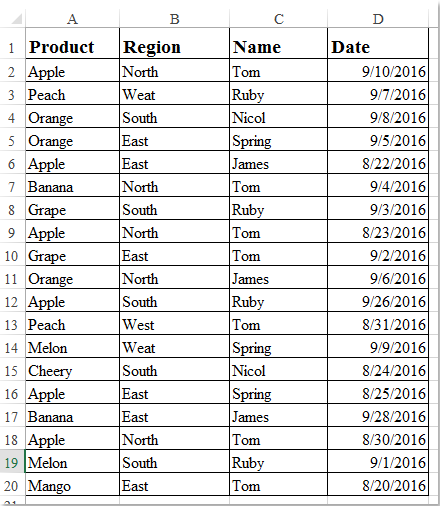Please enter this formula into a blank cell where you want to get the result, G2, for instance:

=SUM(IF("Tom"=\$C\$2:\$C\$20, 1/(COUNTIFS(\$C\$2:\$C\$20, "Tom", \$A\$2:\$A\$20, \$A\$2:\$A\$20)), 0)), and then press Shift + Ctrl + Enter keys together to get the correct result, see screenshot: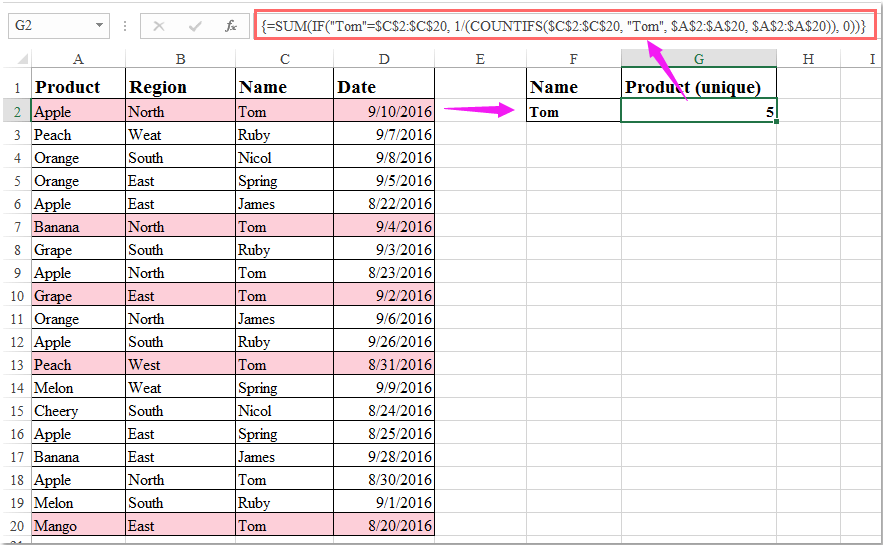Note: In the above formula, “Tom” is the name criteria that you want to count based on, C2:C20 is the cells contains the name criteria, A2:A20 is the cells that you want to count the unique values.

####Count unique values based on two given dates

To calculate the unique values between two given dates, for example, I want to count the unique product between the date range 2016/9/1 and 2016/9/30, please apply this formula:

=SUM(IF(\$D\$2:\$D\$20<=DATE(2016, 9, 30)*(\$D\$2:\$D\$20>=DATE(2016, 9, 1)), 1/COUNTIFS( \$A\$2:\$A\$20, \$A\$2:\$A\$20, \$D\$2:\$D\$20, "<="&DATE(2016, 9, 30),\$D\$2:\$D\$20, ">="&DATE(2016, 9, 1))), 0), and then press Shift + Ctrl + Enter keys together to get the unique result, see screenshot: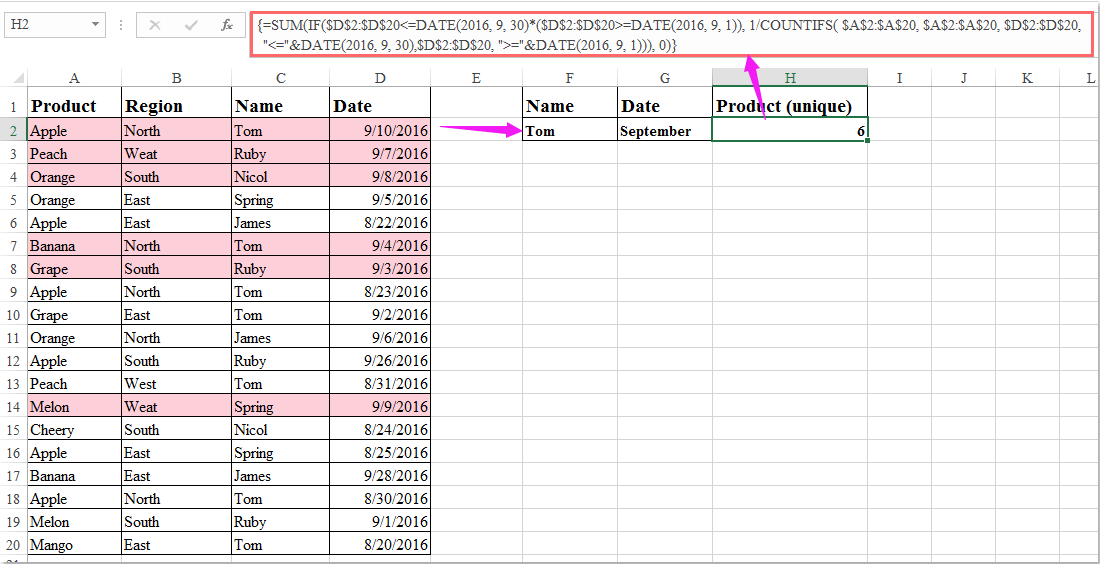Note: In the above formula, the date 2016,9,1 and 2016,9,30 are the start date and end date that you want to count based on, D2:D20 is the cells contain the date criteria, A2:A20 is the cells that you want to count the unique values from.

####Count unique values based on two criteria

If you want to count the unique product that Tom sale in September, the following formula can help you.

Please enter this formula into a blank cell to output the result, H2, for example.

=SUM(IF(("Tom"=\$C\$2:\$C\$20)*(\$D\$2:\$D\$20<=DATE(2016, 9, 30)*(\$D\$2:\$D\$20>=DATE(2016, 9, 1))), 1/COUNTIFS(\$C\$2:\$C\$20, "Tom", \$A\$2:\$A\$20, \$A\$2:\$A\$20, \$D\$2:\$D\$20, "<="&DATE(2016, 9, 30),\$D\$2:\$D\$20, ">="&DATE(2016, 9, 1))), 0) and then press Shift + Ctrl + Enter keys together to get the unique result, see screenshot: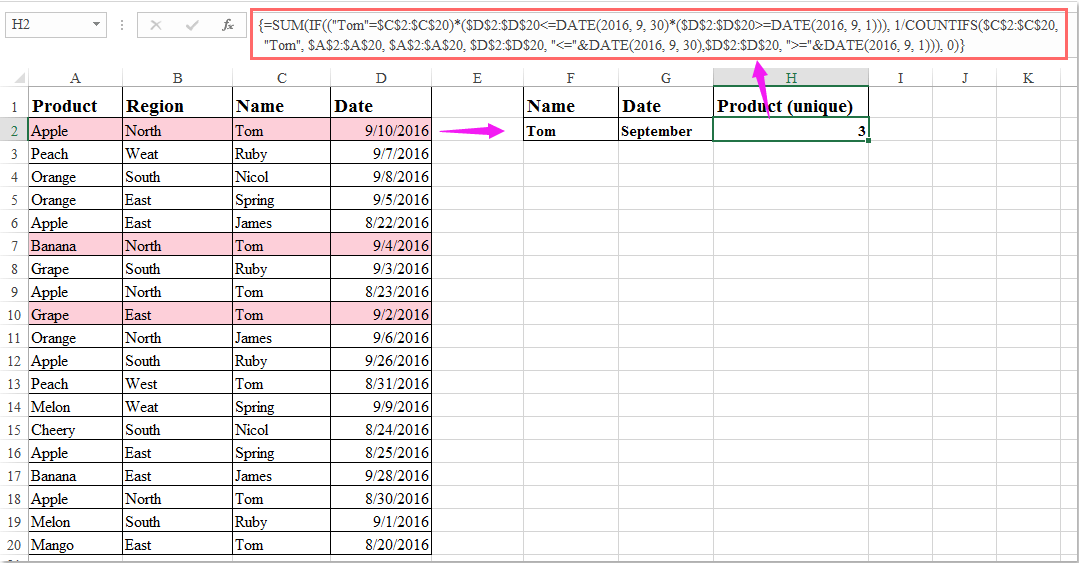Notes:

1. In the above formula, “Tom” is the name criteria, 2016,9,1 and 2016,9,30 are the two dates that you want to count based on, C2:C20 is the cells contains the name criteria, and D2:D20 is the cells contain the date, A2:A20 is the range of cells that you want to count the unique values.

2. If you need to use an “or” criteria to count the unique values, such as, calculate the products which sold by Tom or in South region, please apply this formula:

=SUM(--(FREQUENCY(IF(("Tom"=\$C\$2:\$C\$20)+("South"=\$B\$2:\$B\$20), COUNTIF(\$A\$2:\$A\$20, "<"&\$A\$2:\$A\$20), ""), COUNTIF(\$A\$2:\$A\$20, "<"&\$A\$2:\$A\$20))>0)), and remember to press Shift + Ctrl + Enter keys together to get the unique result, see screenshot: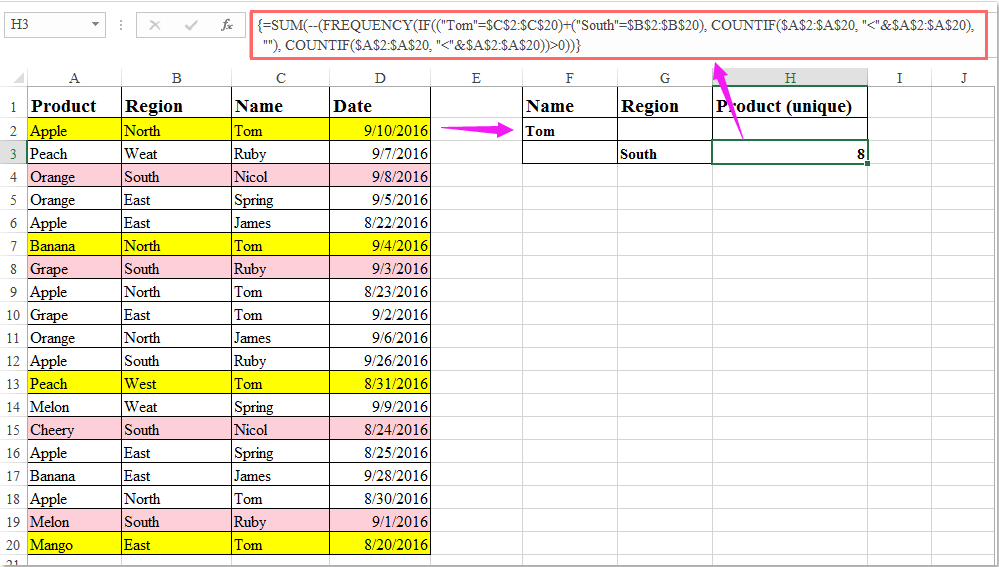####Count unique values based on three criteria

To count the unique product with three criteria, the formula may be more complex. Let’s say, calculating the unique products which are sold by Tom in September and in region North. Please do as this:

Enter this formula into a blank cell to output the result, I2, for example:

=SUM(IF(("Tom"=\$C\$2:\$C\$20)*(\$D\$2:\$D\$20<=DATE(2016, 9, 30))*(\$D\$2:\$D\$20>=DATE(2016, 9, 1))*("North"=\$B\$2:\$B\$20), 1/COUNTIFS(\$C\$2:\$C\$20, "Tom", \$A\$2:\$A\$20, \$A\$2:\$A\$20, \$D\$2:\$D\$20, "<="&DATE(2016, 9, 30), \$D\$2:\$D\$20, ">="&DATE(2016, 9, 1), \$B\$2:\$B\$20, "North")), 0), and then press Shift + Ctrl + Enter keys together to get the unique result, see screenshot: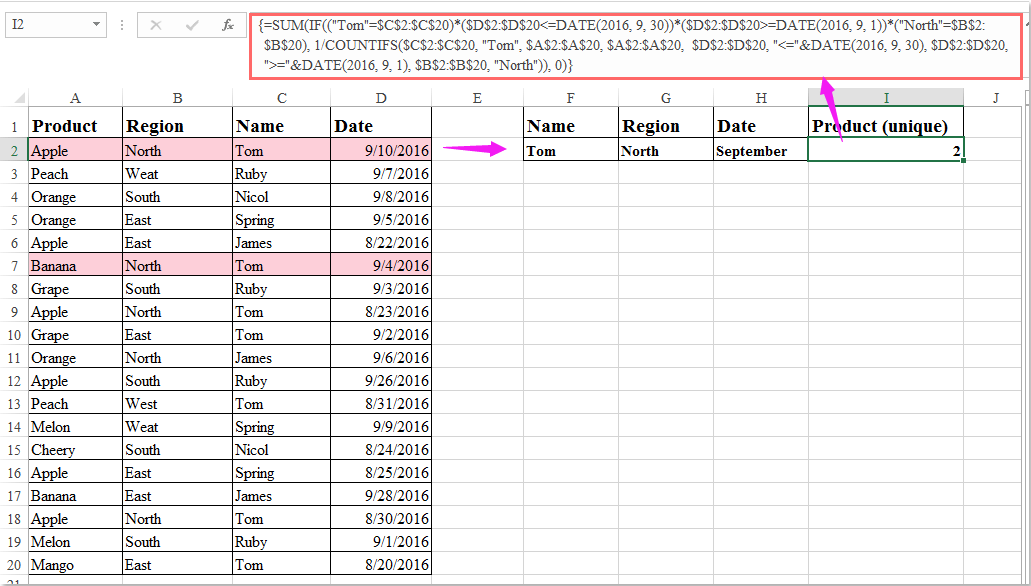### Best Office Productivity Tools

 Popular Features: Find, Highlight or Identify Duplicates   |  Delete Blank Rows   |  Combine Columns or Cells without Losing Data   |   Round without Formula ... Super Lookup: Multiple Criteria VLookup  |   Multiple Value VLookup  |   VLookup Across Multiple Sheets   |   Fuzzy Lookup .... Advanced Drop-down List: Quickly Create Drop Down List   |  Dependent Drop Down List   |  Multi-select Drop Down List .... Column Manager: Add a Specific Number of Columns  |  Move Columns  |  Toggle Visibility Status of Hidden Columns  |  Compare Ranges & Columns ... Featured Features: Grid Focus   |  Design View   |   Big Formula Bar   |  Workbook & Sheet Manager   |  Resource Library (Auto Text)   |  Date Picker   |  Combine Worksheets   |  Encrypt/Decrypt Cells   |  Send Emails by List   |  Super Filter   |   Special Filter (filter bold/italic/strikethrough...) ... Top 15 Toolsets:  12 Text Tools (Add Text, Remove Characters, ...)   |   50+ Chart Types (Gantt Chart, ...)   |   40+ Practical Formulas (Calculate age based on birthday, ...)   |   19 Insertion Tools (Insert QR Code, Insert Picture from Path, ...)   |   12 Conversion Tools (Numbers to Words, Currency Conversion, ...)   |   7 Merge & Split Tools (Advanced Combine Rows, Split Cells, ...)   |   ... and more

Supercharge Your Excel Skills with Kutools for Excel, and Experience Efficiency Like Never Before. Kutools for Excel Offers Over 300 Advanced Features to Boost Productivity and Save Time.  Click Here to Get The Feature You Need The Most...#### Office Tab Brings Tabbed interface to Office, and Make Your Work Much Easier

• Enable tabbed editing and reading in Word, Excel, PowerPoint, Publisher, Access, Visio and Project.
• Open and create multiple documents in new tabs of the same window, rather than in new windows.
• Increases your productivity by 50%, and reduces hundreds of mouse clicks for you every day!No ratings yet. Be the first to rate!
This comment was minimized by the moderator on the site
For all the above formula an you suggest a non array formula as my data runs to 25000 rows. I need a free suggestions and not paid ones
This comment was minimized by the moderator on the site
Hi,
This is great - except I cant get it to work for what I require
I have two sheets - a Summary sheet, and another sheet containing data
The dates are dynamic - so you enter the date ranges in the Summary sheet in two cells (from B2 to D2)
When I replace DATE(2022,6,1) with B2 it comes back with "a value used in the formula is the wrong data type"
When I test with putting DATE(2022,6,1) and DATE (2022,6,30) in the from - to parts in the formula - I get 0 as the result - which is wrong.
Note: I'm in Ireland - so the date format here is dd.mm.yy - changing things doesn't fix - and adds confusion tbh
My formula is
=SUM(IF(Sheet4!\$C\$2:Sheet4!\$C\$65<=(D2)*(Sheet4!\$C\$2:Sheet4!\$C\$65>=(B2)), 1/COUNTIFS(Sheet4!\$A\$2:Sheet4!\$A\$65, Sheet4!\$A\$2:Sheet4!\$A\$65, Sheet4!\$C\$2:Sheet4!\$C\$65, "<="&D2,Sheet4!\$C\$2:Sheet4!\$C\$65, ">="&B2))),0)
Where Sheet4 contains the data, C2:C65 are cells with dates, A2:A65 are cells with project numbers - where there maybe duplicates
Any help - greatly appreciated,
Thanks
This comment was minimized by the moderator on the site
1 month2 brand name 3 executive wise4 mix party nameCount unique party name
This comment was minimized by the moderator on the site
Count Unique Values Based On four Criteria
This comment was minimized by the moderator on the site
I am trying to use this method to calculate unique customers for a particular product (where a customer may have bought multiple times, but I want unique customers). If I enter the formula but limit the range to a subset of just 5 rows that I know contain a duplicate customer, it works fine. But when I apply to the whole column, e.g. \$D:\$D, it calculates endlessly; if it finishes, it returns a wrong result. But now it's not even finishing and I have to end the Excel process. Is this just too costly in terms of CPU to apply to a large volume of data (e.g. 1500 rows)?
This comment was minimized by the moderator on the site

{=SUM(IF(("Regular"='Raw Data'!\$G\$5:\$G\$1785)*('Raw Data'!\$D\$5:\$D\$1785<=DATE(2019,6,30)*('Raw Data'!\$D\$5:\$D\$1785>=DATE(2019,6,1))),1/COUNTIFS('Raw Data'!\$B\$5:\$B\$1785,'Raw Data'!\$B\$5:\$B\$1785,'Raw Data'!\$D\$5:\$D\$1785,"<="&DATE(2019,6,30),'Raw Data'!\$D\$5:\$D\$1785,">="&DATE(2019,6,1))),0)}
This comment was minimized by the moderator on the site
my question.
I mean that filtered rows , and not count hidden rows.
This comment was minimized by the moderator on the site
"if count visible rows."
I mean filtered rows , and not count rows hidden.
This comment was minimized by the moderator on the site